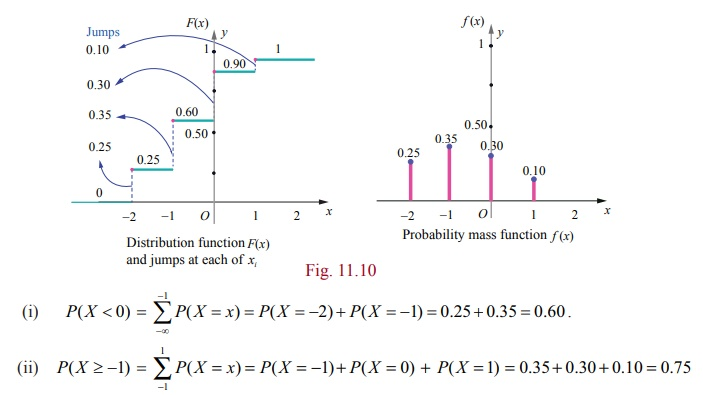Home | | Maths 12th Std | Probability Mass Function from Cumulative Distribution Function

# Probability Mass Function from Cumulative Distribution Function

Here the jump (because of discontinuity) acts as a probability. That is, the set of discontinuities of a cumulative distribution function is at most countable!

## Probability Mass Function from Cumulative Distribution Function

For a discrete random variable X, the cumulative distribution function has jumps at each of the xi , and is constant between successive xi s . The height of the jump at xi fxi ) ; in this way the is probability at xi can be retrieved from F .

Suppose X is a discrete random variable taking the values x1 , x2 , x3 , such that x1 < x2 , < xand F ( xi ) is the distribution function. Then the probability mass function f ( xi ) is given by

(xi) = F (xi ) F (xi-1 ) , = 1, 2, 3, . . .

Note

The jump of a function F ( x) at x = a is |F ( a + )  F ( a  )|. Since F is non-decreasing and continuous to the right, the jump of a cumulative distribution function F is PX = x) = Fx) − Fx ) .

Here the jump (because of discontinuity) acts as a probability. That is, the set of discontinuities of a cumulative distribution function is at most countable!

### Example 11.9

Find the probability mass function f ( x) of the discrete random variable X whose cumulative distribution function F ( x) is given byAlso find (i) P( X < 0) and (ii) P( X ≥ − 1) .

### Solution

Since X is a discrete random variable, from the given data, X takes on the values 2, −1, 0, and 1.

For discrete random variable X, by definition, we have f ( x) = PX = x)

Therefore left hand limit of F(x) at x = −2 is F (  )

f(2) = P(X = − 2) = F(− 2) −  F(− 2   ) = 0.25 − 0 = 0.25.

Similarly for other jump points, we have

(1) P(X = − 1) = F(− 1) −  F(−2) = 0.60 − 0.25 = 0.35.

(0) = P(X = 0) = F(0) −  F(−1) = 0.90 −  0.60 = 0.30 ,

(1) = P( X = 1) = F(1) − F(0) = 1 −  0.90 = 0.10 .

Therefore the probability mass function isThe distribution function F ( x) has jumps at x = − 2, − 1, 0, and 1. The jumps are respectively 0.25, 0.35, 0.30, and 0.1 is shown in the figure given below.

These jumps determine the probability mass functionExample 11.10

A random variable X has the following probability mass function.Find (i) P (2 < X < 6) (ii) P (2 ≤ X < 5) (iii) P( 4) (iv) P(3< X)

Solution

Since the given function is a probability mass function, the total probability is one. That is (x) = 1.

From the given data k + 2k + 6k + 5k + 6k + 10k = 1

30k = 1

k = 1/30

Therefore the probability mass function isTags : Probability Distributions | Mathematics , 12th Maths : UNIT 11 : Probability Distributions
Study Material, Lecturing Notes, Assignment, Reference, Wiki description explanation, brief detail
12th Maths : UNIT 11 : Probability Distributions : Probability Mass Function from Cumulative Distribution Function | Probability Distributions | Mathematics